Publicité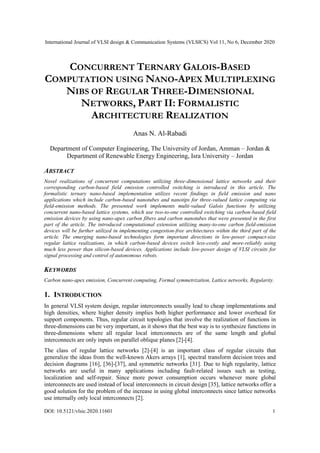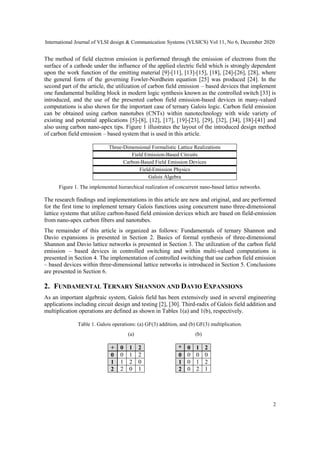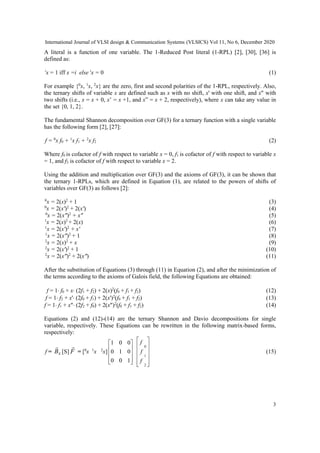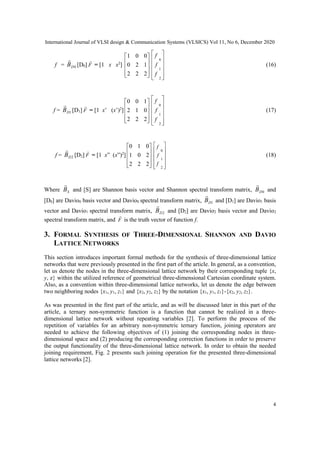Publicité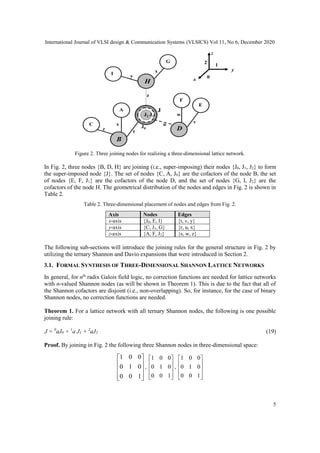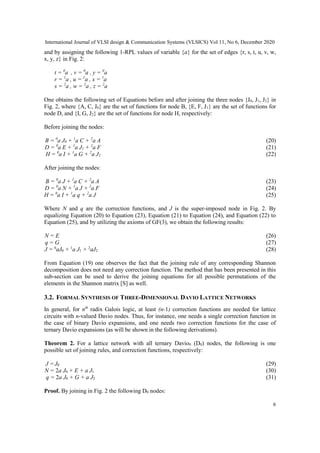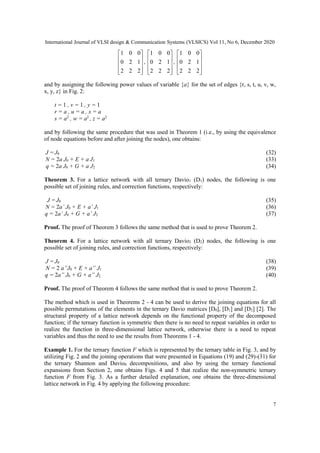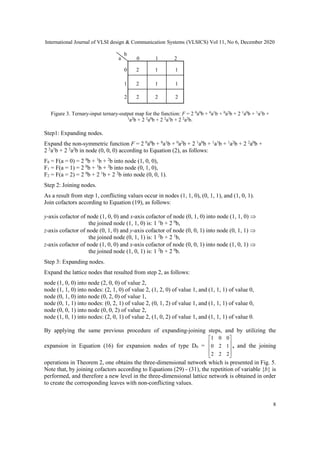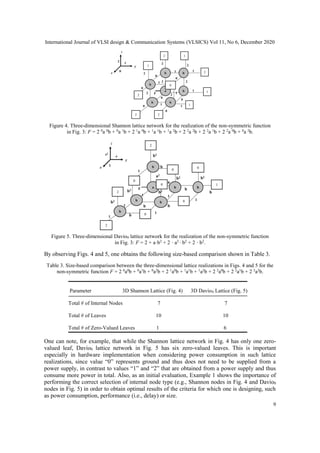Publicité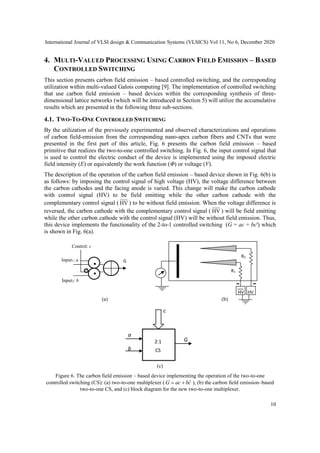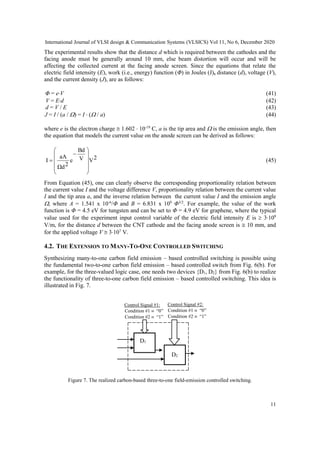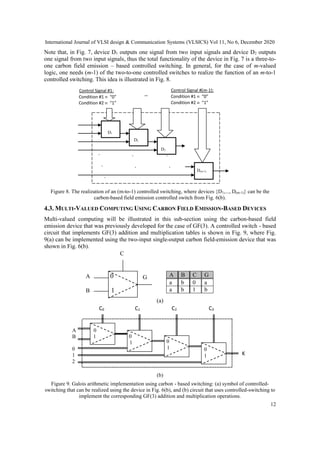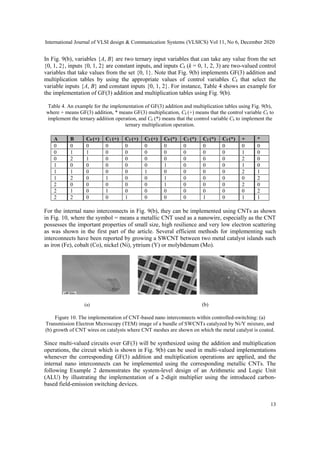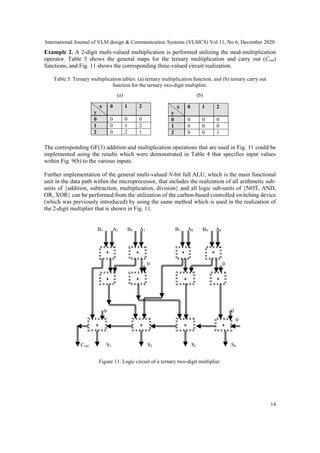Publicité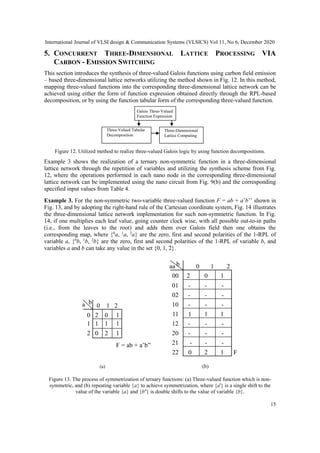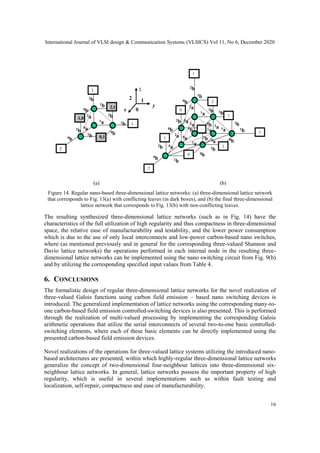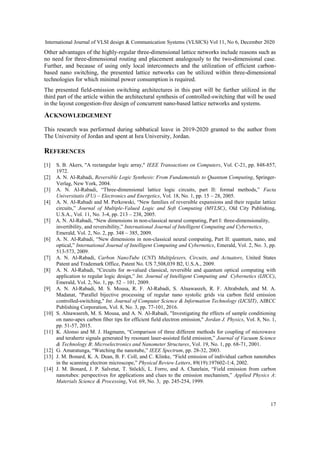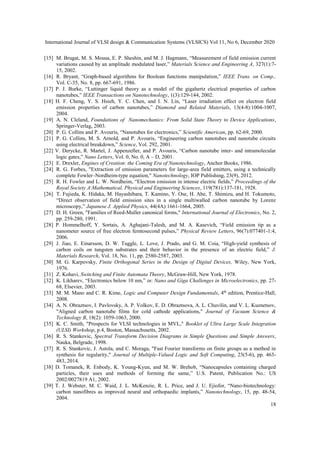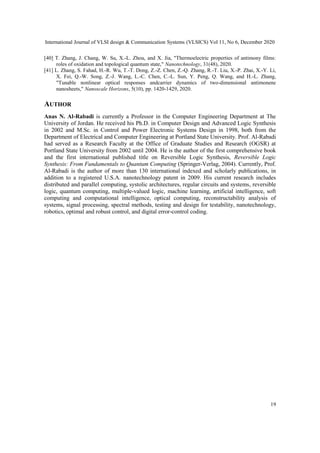Prochain SlideShare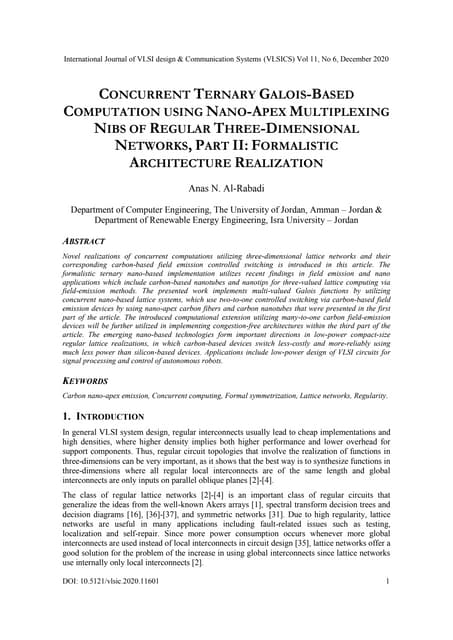Concurrent Ternary Galois-based Computation using Nano-apex Multiplexing Nibs...
Chargement dans ... 3
1 sur 19
Publicité

### CONCURRENT TERNARY GALOIS-BASED COMPUTATION USING NANO-APEX MULTIPLEXING NIBS OF REGULAR THREE-DIMENSIONAL NETWORKS, PART II: FORMALISTIC ARCHITECTURE REALIZATION

1. International Journal of VLSI design & Communication Systems (VLSICS) Vol 11, No 6, December 2020 DOI: 10.5121/vlsic.2020.11601 1 CONCURRENT TERNARY GALOIS-BASED COMPUTATION USING NANO-APEX MULTIPLEXING NIBS OF REGULAR THREE-DIMENSIONAL NETWORKS, PART II: FORMALISTIC ARCHITECTURE REALIZATION Anas N. Al-Rabadi Department of Computer Engineering, The University of Jordan, Amman – Jordan & Department of Renewable Energy Engineering, Isra University – Jordan ABSTRACT Novel realizations of concurrent computations utilizing three-dimensional lattice networks and their corresponding carbon-based field emission controlled switching is introduced in this article. The formalistic ternary nano-based implementation utilizes recent findings in field emission and nano applications which include carbon-based nanotubes and nanotips for three-valued lattice computing via field-emission methods. The presented work implements multi-valued Galois functions by utilizing concurrent nano-based lattice systems, which use two-to-one controlled switching via carbon-based field emission devices by using nano-apex carbon fibers and carbon nanotubes that were presented in the first part of the article. The introduced computational extension utilizing many-to-one carbon field-emission devices will be further utilized in implementing congestion-free architectures within the third part of the article. The emerging nano-based technologies form important directions in low-power compact-size regular lattice realizations, in which carbon-based devices switch less-costly and more-reliably using much less power than silicon-based devices. Applications include low-power design of VLSI circuits for signal processing and control of autonomous robots. KEYWORDS Carbon nano-apex emission, Concurrent computing, Formal symmetrization, Lattice networks, Regularity. 1. INTRODUCTION In general VLSI system design, regular interconnects usually lead to cheap implementations and high densities, where higher density implies both higher performance and lower overhead for support components. Thus, regular circuit topologies that involve the realization of functions in three-dimensions can be very important, as it shows that the best way is to synthesize functions in three-dimensions where all regular local interconnects are of the same length and global interconnects are only inputs on parallel oblique planes -. The class of regular lattice networks - is an important class of regular circuits that generalize the ideas from the well-known Akers arrays , spectral transform decision trees and decision diagrams , -, and symmetric networks . Due to high regularity, lattice networks are useful in many applications including fault-related issues such as testing, localization and self-repair. Since more power consumption occurs whenever more global interconnects are used instead of local interconnects in circuit design , lattice networks offer a good solution for the problem of the increase in using global interconnects since lattice networks use internally only local interconnects .
3. International Journal of VLSI design & Communication Systems (VLSICS) Vol 11, No 6, December 2020 3 A literal is a function of one variable. The 1-Reduced Post literal (1-RPL) , ,  is defined as: i x = 1 iff x =i else i x = 0 (1) For example {0 x, 1 x, 2 x} are the zero, first and second polarities of the 1-RPL, respectively. Also, the ternary shifts of variable x are defined such as x with no shift, x' with one shift, and x" with two shifts (i.e., x = x + 0, x’ = x +1, and x” = x + 2, respectively), where x can take any value in the set {0, 1, 2}. The fundamental Shannon decomposition over GF(3) for a ternary function with a single variable has the following form , : f = 0 x f0 + 1 x f1 + 2 x f2 (2) Where f0 is cofactor of f with respect to variable x = 0, f1 is cofactor of f with respect to variable x = 1, and f2 is cofactor of f with respect to variable x = 2. Using the addition and multiplication over GF(3) and the axioms of GF(3), it can be shown that the ternary 1-RPLs, which are defined in Equation (1), are related to the powers of shifts of variables over GF(3) as follows : 0 x = 2(x)2 + 1 (3) 0 x = 2(x')2 + 2(x') (4) 0 x = 2(x")2 + x" (5) 1 x = 2(x)2 + 2(x) (6) 1 x = 2(x')2 + x' (7) 1 x = 2(x")2 + 1 (8) 2 x = 2(x)2 + x (9) 2 x = 2(x')2 + 1 (10) 2 x = 2(x")2 + 2(x") (11) After the substitution of Equations (3) through (11) in Equation (2), and after the minimization of the terms according to the axioms of Galois field, the following Equations are obtained: f = 1 f0 + x (2f1 + f2) + 2(x)2 (f0 + f1 + f2) (12) f = 1 f2 + x' (2f0 + f1) + 2(x')2 (f0 + f1 + f2) (13) f = 1 f1 + x" (2f2 + f0) + 2(x")2 (f0 + f1 + f2) (14) Equations (2) and (12)-(14) are the ternary Shannon and Davio decompositions for single variable, respectively. These Equations can be rewritten in the following matrix-based forms, respectively: f = S B  [S] F  = [0 x 1 x 2 x]           1 0 0 0 1 0 0 0 1             f f f 2 1 0 (15)
4. International Journal of VLSI design & Communication Systems (VLSICS) Vol 11, No 6, December 2020 4 f = 0 D B  [D0] F  = [1 x x2 ]           2 2 2 1 2 0 0 0 1             f f f 2 1 0 (16) f = 1 D B  [D1] F  = [1 x’ (x’)2 ]           2 2 2 0 1 2 1 0 0             f f f 2 1 0 (17) f = 2 D B  [D2] F  = [1 x” (x”)2 ]           2 2 2 2 0 1 0 1 0             f f f 2 1 0 (18) Where S B  and [S] are Shannon basis vector and Shannon spectral transform matrix, 0 D B  and [D0] are Davio0 basis vector and Davio0 spectral transform matrix, 1 D B  and [D1] are Davio1 basis vector and Davio1 spectral transform matrix, 2 D B  and [D2] are Davio2 basis vector and Davio2 spectral transform matrix, and F  is the truth vector of function f. 3. FORMAL SYNTHESIS OF THREE-DIMENSIONAL SHANNON AND DAVIO LATTICE NETWORKS This section introduces important formal methods for the synthesis of three-dimensional lattice networks that were previously presented in the first part of the article. In general, as a convention, let us denote the nodes in the three-dimensional lattice network by their corresponding tuple {x, y, z} within the utilized reference of geometrical three-dimensional Cartesian coordinate system. Also, as a convention within three-dimensional lattice networks, let us denote the edge between two neighboring nodes {x1, y1, z1} and {x2, y2, z2} by the notation {x1, y1, z1}-{x2, y2, z2}. As was presented in the first part of the article, and as will be discussed later in this part of the article, a ternary non-symmetric function is a function that cannot be realized in a three- dimensional lattice network without repeating variables . To perform the process of the repetition of variables for an arbitrary non-symmetric ternary function, joining operators are needed to achieve the following objectives of (1) joining the corresponding nodes in three- dimensional space and (2) producing the corresponding correction functions in order to preserve the output functionality of the three-dimensional lattice network. In order to obtain the needed joining requirement, Fig. 2 presents such joining operation for the presented three-dimensional lattice networks .
5. International Journal of VLSI design & Communication Systems (VLSICS) Vol 11, No 6, December 2020 5 Figure 2. Three joining nodes for realizing a three-dimensional lattice network. In Fig. 2, three nodes {B, D, H} are joining (i.e., super-imposing) their nodes {J0, J1, J2} to form the super-imposed node {J}. The set of nodes {C, A, J0} are the cofactors of the node B, the set of nodes {E, F, J1} are the cofactors of the node D, and the set of nodes {G, I, J2} are the cofactors of the node H. The geometrical distribution of the nodes and edges in Fig. 2 is shown in Table 2. Table 2. Three-dimensional placement of nodes and edges from Fig. 2. Axis Nodes Edges x-axis {J0, E, I} {t, v, y} y-axis {C, J1, G} {r, u, x} z-axis {A, F, J2} {s, w, z} The following sub-sections will introduce the joining rules for the general structure in Fig. 2 by utilizing the ternary Shannon and Davio expansions that were introduced in Section 2. 3.1. FORMAL SYNTHESIS OF THREE-DIMENSIONAL SHANNON LATTICE NETWORKS In general, for nth radix Galois field logic, no correction functions are needed for lattice networks with n-valued Shannon nodes (as will be shown in Theorem 1). This is due to the fact that all of the Shannon cofactors are disjoint (i.e., non-overlapping). So, for instance, for the case of binary Shannon nodes, no correction functions are needed. Theorem 1. For a lattice network with all ternary Shannon nodes, the following is one possible joining rule: J = 0 aJ0 + 1 a J1 + 2 aJ2 (19) Proof. By joining in Fig. 2 the following three Shannon nodes in three-dimensional space:           1 0 0 0 1 0 0 0 1 ,           1 0 0 0 1 0 0 0 1 ,           1 0 0 0 1 0 0 0 1
6. International Journal of VLSI design & Communication Systems (VLSICS) Vol 11, No 6, December 2020 6 and by assigning the following 1-RPL values of variable {a} for the set of edges {r, s, t, u, v, w, x, y, z} in Fig. 2: t = 0 a , v = 0 a , y = 0 a r = 1 a , u = 1 a , x = 1 a s = 2 a , w = 2 a , z = 2 a One obtains the following set of Equations before and after joining the three nodes {J0, J1, J2} in Fig. 2, where {A, C, J0} are the set of functions for node B, {E, F, J1} are the set of functions for node D, and {I, G, J2} are the set of functions for node H, respectively: Before joining the nodes: B = 0 a J0 + 1 a C + 2 a A (20) D = 0 a E + 1 a J1 + 2 a F (21) H = 0 a I + 1 a G + 2 a J2 (22) After joining the nodes: B = 0 a J + 1 a C + 2 a A (23) D = 0 a N + 1 a J + 2 a F (24) H = 0 a I + 1 a q + 2 a J (25) Where N and q are the correction functions, and J is the super-imposed node in Fig. 2. By equalizing Equation (20) to Equation (23), Equation (21) to Equation (24), and Equation (22) to Equation (25), and by utilizing the axioms of GF(3), we obtain the following results: N = E (26) q = G (27) J = 0 aJ0 + 1 a J1 + 2 aJ2 (28) From Equation (19) one observes the fact that the joining rule of any corresponding Shannon decomposition does not need any correction function. The method that has been presented in this sub-section can be used to derive the joining equations for all possible permutations of the elements in the Shannon matrix [S] as well. 3.2. FORMAL SYNTHESIS OF THREE-DIMENSIONAL DAVIO LATTICE NETWORKS In general, for nth radix Galois logic, at least (n-1) correction functions are needed for lattice circuits with n-valued Davio nodes. Thus, for instance, one needs a single correction function in the case of binary Davio expansions, and one needs two correction functions for the case of ternary Davio expansions (as will be shown in the following derivations). Theorem 2. For a lattice network with all ternary Davio0 (D0) nodes, the following is one possible set of joining rules, and correction functions, respectively: J = J0 (29) N = 2a J0 + E + a J1 (30) q = 2a J0 + G + a J2 (31) Proof. By joining in Fig. 2 the following D0 nodes:
7. International Journal of VLSI design & Communication Systems (VLSICS) Vol 11, No 6, December 2020 7           2 2 2 1 2 0 0 0 1 ,           2 2 2 1 2 0 0 0 1 ,           2 2 2 1 2 0 0 0 1 and by assigning the following power values of variable {a} for the set of edges {r, s, t, u, v, w, x, y, z} in Fig. 2: t = 1 , v = 1 , y = 1 r = a , u = a , x = a s = a2 , w = a2 , z = a2 and by following the same procedure that was used in Theorem 1 (i.e., by using the equivalence of node equations before and after joining the nodes), one obtains: J = J0 (32) N = 2a J0 + E + a J1 (33) q = 2a J0 + G + a J2 (34) Theorem 3. For a lattice network with all ternary Davio1 (D1) nodes, the following is one possible set of joining rules, and correction functions, respectively: J = J0 (35) N = 2a’ J0 + E + a’ J1 (36) q = 2a’ J0 + G + a’ J2 (37) Proof. The proof of Theorem 3 follows the same method that is used to prove Theorem 2. Theorem 4. For a lattice network with all ternary Davio2 (D2) nodes, the following is one possible set of joining rules, and correction functions, respectively: J = J0 (38) N = 2 a”J0 + E + a” J1 (39) q = 2a” J0 + G + a” J2 (40) Proof. The proof of Theorem 4 follows the same method that is used to prove Theorem 2. The method which is used in Theorems 2 - 4 can be used to derive the joining equations for all possible permutations of the elements in the ternary Davio matrices [D0], [D1] and [D2] . The structural property of a lattice network depends on the functional property of the decomposed function; if the ternary function is symmetric then there is no need to repeat variables in order to realize the function in three-dimensional lattice network, otherwise there is a need to repeat variables and thus the need to use the results from Theorems 1 - 4. Example 1. For the ternary function F which is represented by the ternary table in Fig. 3, and by utilizing Fig. 2 and the joining operations that were presented in Equations (19) and (29)-(31) for the ternary Shannon and Davio0 decompositions, and also by using the ternary functional expansions from Section 2, one obtains Figs. 4 and 5 that realize the non-symmetric ternary function F from Fig. 3. As a further detailed explanation, one obtains the three-dimensional lattice network in Fig. 4 by applying the following procedure:
8. International Journal of VLSI design & Communication Systems (VLSICS) Vol 11, No 6, December 2020 8 Figure 3. Ternary-input ternary-output map for the function: F = 2 0 a0 b + 0 a1 b + 0 a2 b + 2 1 a0 b + 1 a1 b + 1 a2 b + 2 2 a0 b + 2 2 a1 b + 2 2 a2 b. Step1: Expanding nodes. Expand the non-symmetric function F = 2 0 a0 b + 0 a1 b + 0 a2 b + 2 1 a0 b + 1 a1 b + 1 a2 b + 2 2 a0 b + 2 2 a1 b + 2 2 a2 b in node (0, 0, 0) according to Equation (2), as follows: F0 = F(a = 0) = 2 0 b + 1 b + 2 b into node (1, 0, 0), F1 = F(a = 1) = 2 0 b + 1 b + 2 b into node (0, 1, 0), F2 = F(a = 2) = 2 0 b + 2 1 b + 2 2 b into node (0, 0, 1). Step 2: Joining nodes. As a result from step 1, conflicting values occur in nodes (1, 1, 0), (0, 1, 1), and (1, 0, 1). Join cofactors according to Equation (19), as follows: y-axis cofactor of node (1, 0, 0) and x-axis cofactor of node (0, 1, 0) into node (1, 1, 0)  the joined node (1, 1, 0) is: 1 1 b + 2 0 b, z-axis cofactor of node (0, 1, 0) and y-axis cofactor of node (0, 0, 1) into node (0, 1, 1)  the joined node (0, 1, 1) is: 1 2 b + 2 1 b, z-axis cofactor of node (1, 0, 0) and x-axis cofactor of node (0, 0, 1) into node (1, 0, 1)  the joined node (1, 0, 1) is: 1 2 b + 2 0 b. Step 3: Expanding nodes. Expand the lattice nodes that resulted from step 2, as follows: node (1, 0, 0) into node (2, 0, 0) of value 2, node (1, 1, 0) into nodes: (2, 1, 0) of value 2, (1, 2, 0) of value 1, and (1, 1, 1) of value 0, node (0, 1, 0) into node (0, 2, 0) of value 1, node (0, 1, 1) into nodes: (0, 2, 1) of value 2, (0, 1, 2) of value 1, and (1, 1, 1) of value 0, node (0, 0, 1) into node (0, 0, 2) of value 2, node (1, 0, 1) into nodes: (2, 0, 1) of value 2, (1, 0, 2) of value 1, and (1, 1, 1) of value 0. By applying the same previous procedure of expanding-joining steps, and by utilizing the expansion in Equation (16) for expansion nodes of type D0 =           2 2 2 1 2 0 0 0 1 , and the joining operations in Theorem 2, one obtains the three-dimensional network which is presented in Fig. 5. Note that, by joining cofactors according to Equations (29) - (31), the repetition of variable {b} is performed, and therefore a new level in the three-dimensional lattice network is obtained in order to create the corresponding leaves with non-conflicting values. a b 0 1 2 0 2 1 1 1 2 1 1 2 2 2 2
9. International Journal of VLSI design & Communication Systems (VLSICS) Vol 11, No 6, December 2020 9 Figure 4. Three-dimensional Shannon lattice network for the realization of the non-symmetric function in Fig. 3: F = 2 0 a 0 b + 0 a 1 b + 2 1 a 0 b + 1 a 1 b + 1 a 2 b + 2 2 a 2 b + 2 2 a 1 b + 2 2 a 0 b + 0 a 2 b. Figure 5. Three-dimensional Davio0 lattice network for the realization of the non-symmetric function in Fig. 3: F = 2 + a·b2 + 2 · a2 · b2 + 2 · b2 . By observing Figs. 4 and 5, one obtains the following size-based comparison shown in Table 3. Table 3. Size-based comparison between the three-dimensional lattice realizations in Figs. 4 and 5 for the non-symmetric function F = 2 0 a0 b + 0 a1 b + 0 a2 b + 2 1 a0 b + 1 a1 b + 1 a2 b + 2 2 a0 b + 2 2 a1 b + 2 2 a2 b. Parameter 3D Shannon Lattice (Fig. 4) 3D Davio0 Lattice (Fig. 5) Total # of Internal Nodes 7 7 Total # of Leaves 10 10 Total # of Zero-Valued Leaves 1 6 One can note, for example, that while the Shannon lattice network in Fig. 4 has only one zero- valued leaf, Davio0 lattice network in Fig. 5 has six zero-valued leaves. This is important especially in hardware implementation when considering power consumption in such lattice realizations, since value “0” represents ground and thus does not need to be supplied from a power supply, in contrast to values “1” and “2” that are obtained from a power supply and thus consume more power in total. Also, as an initial evaluation, Example 1 shows the importance of performing the correct selection of internal node type (e.g., Shannon nodes in Fig. 4 and Davio0 nodes in Fig. 5) in order to obtain optimal results of the criteria for which one is designing, such as power consumption, performance (i.e., delay) or size.
10. International Journal of VLSI design & Communication Systems (VLSICS) Vol 11, No 6, December 2020 10 4. MULTI-VALUED PROCESSING USING CARBON FIELD EMISSION – BASED CONTROLLED SWITCHING This section presents carbon field emission – based controlled switching, and the corresponding utilization within multi-valued Galois computing . The implementation of controlled switching that use carbon field emission – based devices within the corresponding synthesis of three- dimensional lattice networks (which will be introduced in Section 5) will utilize the accumulative results which are presented in the following three sub-sections. 4.1. TWO-TO-ONE CONTROLLED SWITCHING By the utilization of the previously experimented and observed characterizations and operations of carbon field-emission from the corresponding nano-apex carbon fibers and CNTs that were presented in the first part of this article, Fig. 6 presents the carbon field emission – based primitive that realizes the two-to-one controlled switching. In Fig. 6, the input control signal that is used to control the electric conduct of the device is implemented using the imposed electric field intensity (E) or equivalently the work function (Φ) or voltage (V). The description of the operation of the carbon field emission – based device shown in Fig. 6(b) is as follows: by imposing the control signal of high voltage (HV), the voltage difference between the carbon cathodes and the facing anode is varied. This change will make the carbon cathode with control signal (HV) to be field emitting while the other carbon cathode with the complementary control signal ( V H ) to be without field emission. When the voltage difference is reversed, the carbon cathode with the complementary control signal ( V H ) will be field emitting while the other carbon cathode with the control signal (HV) will be without field emission. Thus, this device implements the functionality of the 2-to-1 controlled switching (G = ac + bc') which is shown in Fig. 6(a). (a) (b) (c) Figure 6. The carbon field emission – based device implementing the operation of the two-to-one controlled switching (CS): (a) two-to-one multiplexer ( c b ac G   ), (b) the carbon field emission–based two-to-one CS, and (c) block diagram for the new two-to-one multiplexer. a b c 2:1 CS G . . Control: c Input1: a Input2: b G HV HV - - R1 R2
11. International Journal of VLSI design & Communication Systems (VLSICS) Vol 11, No 6, December 2020 11 The experimental results show that the distance d which is required between the cathodes and the facing anode must be generally around 10 mm, else beam distortion will occur and will be affecting the collected current at the facing anode screen. Since the equations that relate the electric field intensity (E), work (i.e., energy) function (Φ) in Joules (J), distance (d), voltage (V), and the current density (J), are as follows: Φ = eV (41) V = Ed (42) d = V / E (43) J = I / (a / ) = I  ( / a) (44) where e is the electron charge  1.602  10-19 C, a is the tip area and  is the emission angle, then the equation that models the current value on the anode screen can be derived as follows: 2 V V Bd e 2 Ωd aA I               (45) From Equation (45), one can clearly observe the corresponding proportionality relation between the current value I and the voltage difference V, proportionality relation between the current value I and the tip area a, and the inverse relation between the current value I and the emission angle , where A = 1.541 x 10-6 /Φ and B = 6.831 x 109 Φ3/2 . For example, the value of the work function is Φ = 4.5 eV for tungsten and can be set to Φ = 4.9 eV for graphene, where the typical value used for the experiment input control variable of the electric field intensity E is  3109 V/m, for the distance d between the CNT cathode and the facing anode screen is  10 mm, and for the applied voltage V  3107 V. 4.2. THE EXTENSION TO MANY-TO-ONE CONTROLLED SWITCHING Synthesizing many-to-one carbon field emission – based controlled switching is possible using the fundamental two-to-one carbon field emission – based controlled switch from Fig. 6(b). For example, for the three-valued logic case, one needs two devices {D1, D2} from Fig. 6(b) to realize the functionality of three-to-one carbon field emission – based controlled switching. This idea is illustrated in Fig. 7. Figure 7. The realized carbon-based three-to-one field-emission controlled switching. Control Signal #1: Condition #1  “0” Condition #2  “1” Control Signal #2: Condition #1  “0” Condition #2  “1” D1 D2
12. International Journal of VLSI design & Communication Systems (VLSICS) Vol 11, No 6, December 2020 12 Note that, in Fig. 7, device D1 outputs one signal from two input signals and device D2 outputs one signal from two input signals, thus the total functionality of the device in Fig. 7 is a three-to- one carbon field emission – based controlled switching. In general, for the case of m-valued logic, one needs (m-1) of the two-to-one controlled switches to realize the function of an m-to-1 controlled switching. This idea is illustrated in Fig. 8. Figure 8. The realization of an (m-to-1) controlled switching, where devices {D1,..., D(m-1)} can be the carbon-based field emission controlled switch from Fig. 6(b). 4.3. MULTI-VALUED COMPUTING USING CARBON FIELD EMISSION-BASED DEVICES Multi-valued computing will be illustrated in this sub-section using the carbon-based field emission device that was previously developed for the case of GF(3). A controlled switch - based circuit that implements GF(3) addition and multiplication tables is shown in Fig. 9, where Fig. 9(a) can be implemented using the two-input single-output carbon field-emission device that was shown in Fig. 6(b). (a) (b) Figure 9. Galois arithmetic implementation using carbon - based switching: (a) symbol of controlled- switching that can be realized using the device in Fig. 6(b), and (b) circuit that uses controlled-switching to implement the corresponding GF(3) addition and multiplication operations. A B C G a b 0 a a b 1 b A 0 B 1 C G . . . D1 Control Signal #1: Condition #1  “0” Condition #2  “1” Control Signal #(m-1): Condition #1  “0” Condition #2  “1” … . . . . . . D2 D3 D(m-1) A 0 B 1 0 1 2 C0 C1 C2 C3 0 1 0 1 K 0 1
13. International Journal of VLSI design & Communication Systems (VLSICS) Vol 11, No 6, December 2020 13 In Fig. 9(b), variables {A, B} are two ternary input variables that can take any value from the set {0, 1, 2}, inputs {0, 1, 2} are constant inputs, and inputs Ck (k = 0, 1, 2, 3) are two-valued control variables that take values from the set {0, 1}. Note that Fig. 9(b) implements GF(3) addition and multiplication tables by using the appropriate values of control variables Ck that select the variable inputs {A, B} and constant inputs {0, 1, 2}. For instance, Table 4 shows an example for the implementation of GF(3) addition and multiplication tables using Fig. 9(b). Table 4. An example for the implementation of GF(3) addition and multiplication tables using Fig. 9(b), where + means GF(3) addition, * means GF(3) multiplication, Ck (+) means that the control variable Ck to implement the ternary addition operation, and Ck (*) means that the control variable Ck to implement the ternary multiplication operation. For the internal nano interconnects in Fig. 9(b), they can be implemented using CNTs as shown in Fig. 10, where the symbol = means a metallic CNT used as a nanowire, especially as the CNT possesses the important properties of small size, high resilience and very low electron scattering as was shown in the first part of the article. Several efficient methods for implementing such interconnects have been reported by growing a SWCNT between two metal catalyst islands such as iron (Fe), cobalt (Co), nickel (Ni), yttrium (Y) or molybdenum (Mo). (a) (b) Figure 10. The implementation of CNT-based nano interconnects within controlled-switching: (a) Transmission Electron Microscopy (TEM) image of a bundle of SWCNTs catalyzed by Ni/Y mixture, and (b) growth of CNT wires on catalysts where CNT meshes are shown on which the metal catalyst is coated. Since multi-valued circuits over GF(3) will be synthesized using the addition and multiplication operations, the circuit which is shown in Fig. 9(b) can be used in multi-valued implementations whenever the corresponding GF(3) addition and multiplication operations are applied, and the internal nano interconnects can be implemented using the corresponding metallic CNTs. The following Example 2 demonstrates the system-level design of an Arithmetic and Logic Unit (ALU) by illustrating the implementation of a 2-digit multiplier using the introduced carbon- based field-emission switching devices. A B C0 (+) C1 (+) C2 (+) C3 (+) C0 (*) C1 (*) C2 (*) C3 (*) + * 0 0 0 0 0 0 0 0 0 0 0 0 0 1 1 0 0 0 0 0 0 0 1 0 0 2 1 0 0 0 0 0 0 0 2 0 1 0 0 0 0 0 1 0 0 0 1 0 1 1 0 0 0 1 0 0 0 0 2 1 1 2 0 1 0 0 1 0 0 0 0 2 2 0 0 0 0 0 1 0 0 0 2 0 2 1 0 1 0 0 0 0 0 0 0 2 2 2 0 0 1 0 0 0 1 0 1 1
14. International Journal of VLSI design & Communication Systems (VLSICS) Vol 11, No 6, December 2020 14 Example 2. A 2-digit multi-valued multiplication is performed utilizing the mod-multiplication operator. Table 5 shows the general maps for the ternary multiplication and carry out (Cout) functions, and Fig. 11 shows the corresponding three-valued circuit realization. Table 5. Ternary multiplication tables: (a) ternary multiplication function, and (b) ternary carry out function for the ternary two-digit multiplier. (a) (b) The corresponding GF(3) addition and multiplication operations that are used in Fig. 11 could be implemented using the results which were demonstrated in Table 4 that specifies input values within Fig. 9(b) to the various inputs. Further implementation of the general multi-valued N-bit full ALU, which is the main functional unit in the data path within the microprocessor, that includes the realization of all arithmetic sub- units of {addition, subtraction, multiplication, division} and all logic sub-units of {NOT, AND, OR, XOR} can be performed from the utilization of the carbon-based controlled switching device (which was previously introduced) by using the same method which is used in the realization of the 2-digit multiplier that is shown in Fig. 11. Figure 11. Logic circuit of a ternary two-digit multiplier. x y 0 1 2 0 0 0 0 1 0 1 2 2 0 2 1 x y 0 1 2 0 0 0 0 1 0 0 0 2 0 0 1 0 * + B0 A0 * + B1 A0 0 + + + + 0 0 Cout S3 S2 S1 S0 * + B0 A1 * + B1 A1 0
15. International Journal of VLSI design & Communication Systems (VLSICS) Vol 11, No 6, December 2020 15 5. CONCURRENT THREE-DIMENSIONAL LATTICE PROCESSING VIA CARBON - EMISSION SWITCHING This section introduces the synthesis of three-valued Galois functions using carbon field emission – based three-dimensional lattice networks utilizing the method shown in Fig. 12. In this method, mapping three-valued functions into the corresponding three-dimensional lattice network can be achieved using either the form of function expression obtained directly through the RPL-based decomposition, or by using the function tabular form of the corresponding three-valued function. Figure 12. Utilized method to realize three-valued Galois logic by using function decompositions. Example 3 shows the realization of a ternary non-symmetric function in a three-dimensional lattice network through the repetition of variables and utilizing the synthesis scheme from Fig. 12, where the operations performed in each nano node in the corresponding three-dimensional lattice network can be implemented using the nano circuit from Fig. 9(b) and the corresponding specified input values from Table 4. Example 3. For the non-symmetric two-variable three-valued function F = ab + a’b’’ shown in Fig. 13, and by adopting the right-hand rule of the Cartesian coordinate system, Fig. 14 illustrates the three-dimensional lattice network implementation for such non-symmetric function. In Fig. 14, if one multiplies each leaf value, going counter clock wise, with all possible out-to-in paths (i.e., from the leaves to the root) and adds them over Galois field then one obtains the corresponding map, where {0 a, 1 a, 2 a} are the zero, first and second polarities of the 1-RPL of variable a, {0 b, 1 b, 2 b} are the zero, first and second polarities of the 1-RPL of variable b, and variables a and b can take any value in the set {0, 1, 2}. (a) (b) Figure 13. The process of symmetrization of ternary functions: (a) Three-valued function which is non- symmetric, and (b) repeating variable {a} to achieve symmetrization, where {a'} is a single shift to the value of the variable {a} and {b''} is double shifts to the value of variable {b}. Three-Valued Tabular Decomposition Three-Dimensional Lattice Computing Galois Three-Valued Function Expression
16. International Journal of VLSI design & Communication Systems (VLSICS) Vol 11, No 6, December 2020 16 (a) (b) Figure 14. Regular nano-based three-dimensional lattice networks: (a) three-dimensional lattice network that corresponds to Fig. 13(a) with conflicting leaves (in dark boxes), and (b) the final three-dimensional lattice network that corresponds to Fig. 13(b) with non-conflicting leaves. The resulting synthesized three-dimensional lattice networks (such as in Fig. 14) have the characteristics of the full utilization of high regularity and thus compactness in three-dimensional space, the relative ease of manufacturability and testability, and the lower power consumption which is due to the use of only local interconnects and low-power carbon-based nano switches, where (as mentioned previously and in general for the corresponding three-valued Shannon and Davio lattice networks) the operations performed in each internal node in the resulting three- dimensional lattice networks can be implemented using the nano switching circuit from Fig. 9(b) and by utilizing the corresponding specified input values from Table 4. 6. CONCLUSIONS The formalistic design of regular three-dimensional lattice networks for the novel realization of three-valued Galois functions using carbon field emission – based nano switching devices is introduced. The generalized implementation of lattice networks using the corresponding many-to- one carbon-based field emission controlled-switching devices is also presented. This is performed through the realization of multi-valued processing by implementing the corresponding Galois arithmetic operations that utilize the serial interconnects of several two-to-one basic controlled- switching elements, where each of these basic elements can be directly implemented using the presented carbon-based field emission devices. Novel realizations of the operations for three-valued lattice systems utilizing the introduced nano- based architectures are presented, within which highly-regular three-dimensional lattice networks generalize the concept of two-dimensional four-neighbour lattices into three-dimensional six- neighbour lattice networks. In general, lattice networks possess the important property of high regularity, which is useful in several implementations such as within fault testing and localization, self-repair, compactness and ease of manufacturability.